DraftKings Make Playoffs Odds
-1000
0.1 to 1Chiefs
87.2% implied probability

-900
0.11 to 1Buccaneers
85.4% implied probability

-300
0.33 to 1Bills
71.2% implied probability

-250
0.4 to 1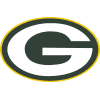Packers
68.2% implied probability

No changes have been recorded yet.
-250
0.4 to 1Rams
68.2% implied probability

-225
0.44 to 149ers
65.6% implied probability

-200
0.5 to 1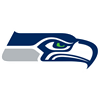Seahawks
63.0% implied probability

-175
0.57 to 1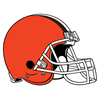Browns
58.9% implied probability

-175
0.57 to 1Saints
60.4% implied probability

-150
0.67 to 1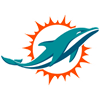Dolphins
56.9% implied probability

-140
0.71 to 1Chargers
55.6% implied probability

-125
0.8 to 1Titans
53.2% implied probability

-125
0.8 to 1Ravens
53.2% implied probability

-120
0.83 to 1Cowboys
52.2% implied probability

-120
0.83 to 1Broncos
52.2% implied probability

+100
1 to 1Cardinals
47.8% implied probability

+110
1.1 to 1Steelers
45.7% implied probability

+120
1.2 to 1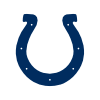Colts
43.1% implied probability

+125
1.25 to 1Patriots
41.7% implied probability

+175
1.75 to 1Football Team
34.4% implied probability

+190
1.9 to 1Vikings
32.6% implied probability

+190
1.9 to 1Eagles
32.6% implied probability

+210
2.1 to 1Panthers
31.1% implied probability

+300
3 to 1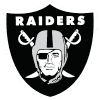Raiders
23.8% implied probability

+300
3 to 1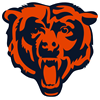Bears
23.8% implied probability

+400
4 to 1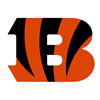Bengals
19.4% implied probability

+500
5 to 1Falcons
15.8% implied probability

+500
5 to 1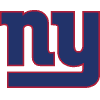Giants
15.9% implied probability

+650
6.5 to 1Jaguars
12.8% implied probability

+700
7 to 1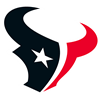Texans
12.0% implied probability

+900
9 to 1Jets
9.6% implied probability

+900
9 to 1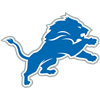Lions
9.5% implied probability